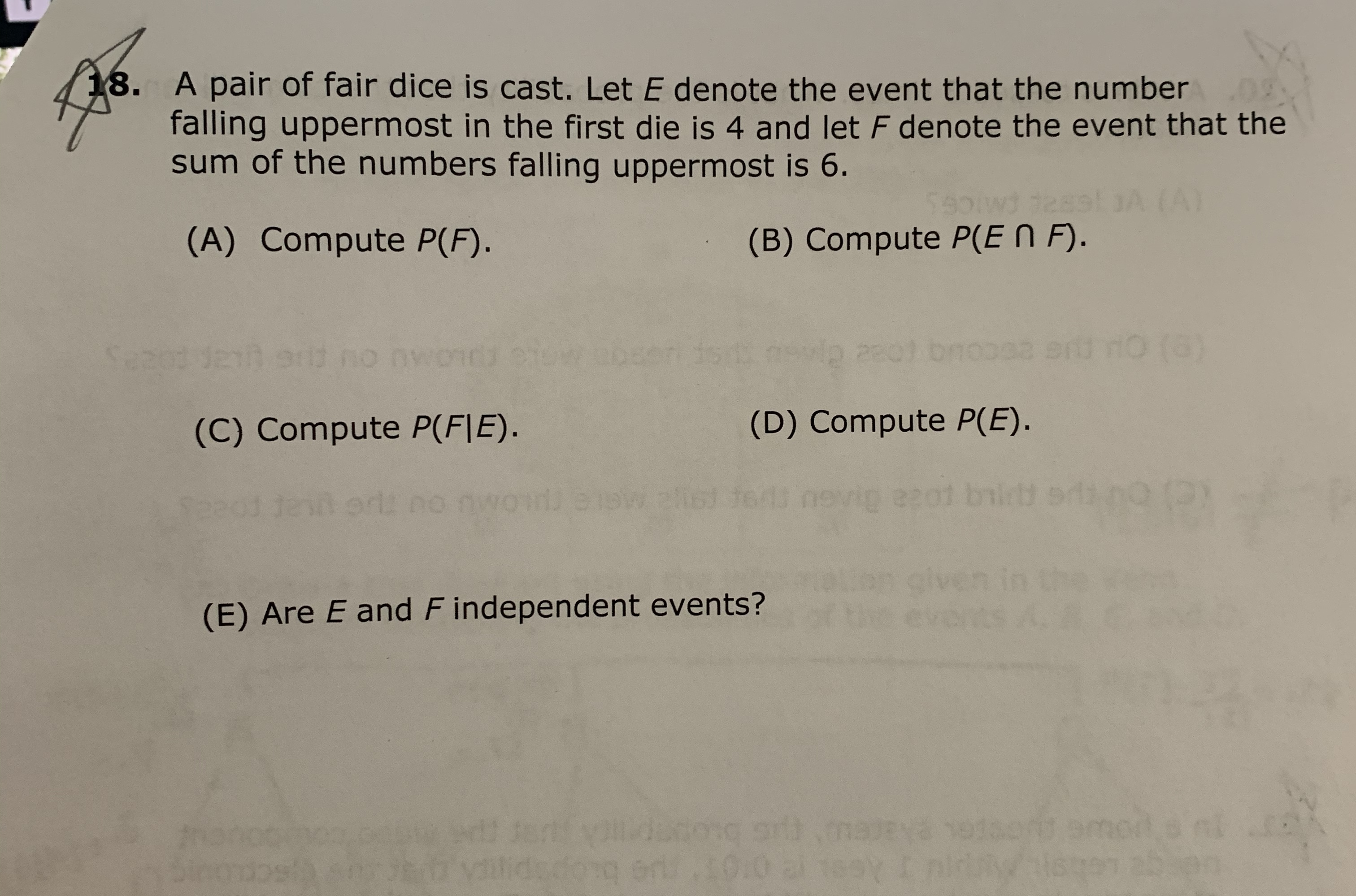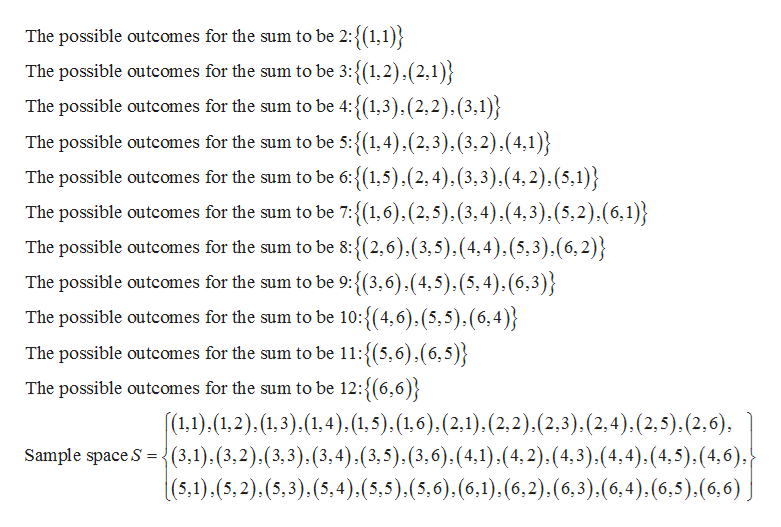# 18. A pair of fair dice is cast. Let E denote the event that the numberfalling uppermost in the first die is 4 and let F denote the event that thesum of the numbers falling uppermost is 6.(A)59(B) Compute P(EN F).12(A) Compute P(F).10 (0)Sabono nv(D) Compute P(E).(C) Compute P(F|E).ne 2yez01 bilrteiWsst(E) Are E and F independent events?ont Bmod25 poudong sid)d:do eri 0.0 al 1eyodosh

Questionhelp_outlineImage Transcriptionclose18. A pair of fair dice is cast. Let E denote the event that the number falling uppermost in the first die is 4 and let F denote the event that the sum of the numbers falling uppermost is 6. (A) 59 (B) Compute P(EN F). 12 (A) Compute P(F). 10 (0) Sabo no nv (D) Compute P(E). (C) Compute P(F|E). ne 2y ez01 bilrt ei Wsst (E) Are E and F independent events? ont Bmod 25 po udong sid) d:do eri 0.0 al 1ey odosh fullscreen
check_circle

Step 1

Note:

Thank you for the question. As your question has more than 3 parts, according to our policy, we are answering the first 3 parts for you. If you need help with any other particular part, please re-post the question and mention the part you need help with.

Step 2

Random experiment of rolling a pair of dice:

Sample space:

The set of all possible outcomes of a probability experiment is called sample space of the experiment.

Here, two fair dice are rolled.

The total number of outcomes in the sample space be n(S) = 62 = 36. That is, there will be 36 equally likely outcomes.

Outcomes will be occurred in ordered pairs. First number in each ordered pair represents the number on the first die and second number in each ordered pair represents the number on the second die. Each of the two numbers can take values 1 to 6.

The sum of the numbers on the dice will be 2, 3, 4, 5, 6, 7, 8, 9, 10 and 11.

The sample space for the experiment is given below:help_outlineImage TranscriptioncloseThe possible outcomes for the sum to be 2:(1,1) The possible outcomes for the sum to be 3:{(1,2).(2,1 The possible outcomes for the sum to be 4:{(1,3),(2,2),(3,1)} The possible outcomes for the sum to be 5:{1,4),(2,3).(3,2).(4,1) The possible outcomes for the sum to be 6:{(1,5).(2,4),(3,3).(4, 2), (5,1)} The possible outcomes for the sum to be 7:{(1,6),(2,5),(3,4),(4,3),(5,2).(6,1)} The possible outcomes for the sum to be 8:{(2,6),(3,5),(4,4). (5,3).(6,2)} The possible outcomes for the sum to be 9:{(3,6),(4,5).(5,4), (6,3) The possible outcomes for the sum to be 10:{(4,6),(5,5).(6,4) The possible outcomes for the sum to be 11:{(5,6).(6,5)} The possible outcomes for the sum to be 12:{(6,6) (1.1),(1,2).(1,3)(1,4),(1.5).(16). (2,1), (2,2).(2,3), (2,4).(2,5). (2,6), Sample space S (3,1), (3,2),(3,3), (3,4),(3,5),(3,6). (4,1) (4, 2).(4,3).(4,4),(4,5).(4,6); |(5,1).(5,2).(5,3),(5,4).(5,5).(5,6).(6,1). (6,2),(6,3).(6,4), (6,5),(6,6) fullscreen
Step 3

A)Calculating P(F):

Event E denotes that the number falling uppermost in the first die is 4 and F denote that the sum of...

### Want to see the full answer?

See Solution

#### Want to see this answer and more?

Solutions are written by subject experts who are available 24/7. Questions are typically answered within 1 hour.*

See Solution
*Response times may vary by subject and question.
Tagged in

### Other